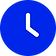gtag('config', 'AW-311446225');# Demand Forecasting - White Papers## objective

The objective of this project was to forecast the future quarterly revenues of one of the leading CPG companies in the United States. The forecast horizon for this project was Q2, Q3, and Q4 of 2020. The underlying value of revenue forecasting stems from the ability to budget expenses more deliberately, plan for growth, improve production scheduling, understand customer behavior, and improve cash flow and credit management.

## forecasting process and implementation

DATA

To carry out our objective, we extracted the company’s quarterly revenue data from Q1 of 1985 to Q1 of 2020, resulting in a total of 141 evenly spaced and sequential observations. In addition, we also extracted the quarterly revenue data of the company’s two main competitors for the same time period. The reason for this was to use the data of the other companies as inputs in our time series regression models.

PREPROCESSING

Since our dataset was extracted in a way that ensured it had no missing values, we did not have to overcome the obstacle of handling missing values in our data. It is crucial to have a dataset without missing values, especially for forecasting, as time series algorithms tend to function poorly with datasets that contain missing values.

However, we had to perform a few preprocessing steps to make the dataset usable for our time series algorithms. For instance, we sorted the records such that the first row contained the oldest observation and the last row contained the newest observation, as this step is necessary for fitting and forecasting.

TIME SERIES ELEMENTS

The blue line in the time series plot above represent our target company’s revenue over time. The green and red lines correspond with the revenues of the company’s two competitors. The sales data for all three companies have a general upward trend. Furthermore, it seems as though their quarterly sales are not seasonal. These conclusions are consistent with the following correlogram of ACFs of our target company. Note that the other two correlograms were almost identical in shape and magnitude.

We can infer that the time series has a trend element, since the ACFs are close to 1 at the start, and they gradually decrease towards 0. In addition, since there are no spikes at multiples of 4 time lags (quarterly), we can conclude that there is no seasonality in this time series.

MODEL BUILDING

After splitting the dataset into a training set with the first 80% of the observations and a testing set with last 20% of the observations, we built a total of 38 forecasting models on the training data. We used the following algorithms to build the forecasting models:

• Naïve methods: naïve model, seasonal naïve model, and naïve model with drift

• Smoothing methods: simple average model, exponential smoothing model with optimal parameters and initial forecast values that minimize SSR, exponential smoothing models with tuned hyperparameter values of alpha, Holt-Winter’s multiplicative model, Holt-Winter’s additive model, and Holt method

• ARIMA Models: non-seasonal ARIMA(p,d,q) models fit with exhaustive search and rough search

• Linear Regression models: linear regression models with all possible combinations of competitor sales as inputs

• Linear Regression models with lagged predictors: linear regression models with all possible combinations of predictors lagged 1 quarter and 2 quarters for the two competitors

• Non-linear Regression models: after performing five non-linear transformations on each predictor (1/predictor, log(predictor), sqrt(predictor), predictor^2, and log-log exponential), we created non-linear regression models using each transformation on its own. We also combined the best transformation for each predictor.

MODEL EVALUATION

After building the models on the training set, we used the models to forecast the observations in the testing set. Subsequently, we evaluated the forecast values using five accuracy measures: Mean Error (ME), Root Mean Square Error (RMSE), Mean Absolute Error (MAE), Mean Percent Error (MPE), and Mean Absolute Percent Error (MAPE). The results are as follows:

The best model was the non-linear regression model that used 1/Competitor#2 as the predictor variable. This model had a MAPE of 3.18%, implying an accuracy of 96.82%. The second-best model was the exponential smoothing model with optimal parameters, which yielded a MAPE of 4.35% (95.65% accuracy).

FUTURE FORECASTS

We analyzed our top two models and found that it is not practical to use a regression model that does not have lagged predictors to make future predictions. This is because such a regression model would require us to forecast future revenues of our competitors and then use the forecasts as inputs to predict our company’s future revenue. In other words, a forecast of our company’s Q2 sales using the best non-linear regression model would require our competitor’s Q2 sales as an input. As the additional layer of forecasting adds extra uncertainty and variability, we decided that it is better to use the second-best model: exponential smoothing with optimal parameters. This choice is justified by the negligible difference between the two models’ accuracies.

Accordingly, we rebuilt the algorithm on the entire dataset, thereby using all the observations (not just training or testing set), and computed the forecasts for the next three quarters. The graph below shows the original time series in black, the fitted time series in red, the forecasts for the next three quarters, and the confidence intervals for the forecasts (80% and 95%).

As seen in the figure above, the exponential smoothing model did an excellent job at fitting the historical data. It is important to note that this model gives the same forecast for the entire forecast horizon because of the way the algorithm is built. Therefore, for accurate results, we would have to update the model each quarter to give the next quarter’s sale forecasts.

Finally, as this model had a 95.65% accuracy while forecasting the sales from Q1 of 2013 to Q1 of 2020 (testing set), we can expect this model to perform similarly in the future.